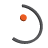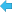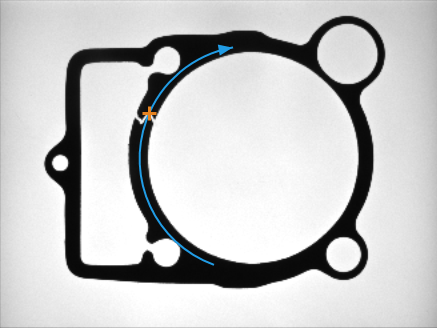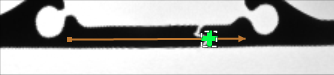Back to Adaptive Vision Studio website

You are here: Start » Filter Reference » Geometry 2D Spatial Transforms » PointAlongArc# PointAlongArc

Module: FoundationLite

Transforms a point to a coordinate system in which the 'axis' arc is vertical or horizontal.

Name Type DescriptioninPoint Point2D Input pointinAxis Arc2D Input axis arcinAxisType Axis Type of axis the input axis arc is parallel toinAxisCoordinate Real Coordinate of the axis arcinInverse Bool Switches to the inverse operationoutPoint Point2D Transformed point

### Applications

Usually used to revert an ImageAlongArc transformation.

### Description

This operation transforms a point - inPoint - between two coordinate systems: the one linked with 'axis' arc inAxis and the original one. Direction of this conversion is based on inInverse value:

• if it is true, inPoint is converted to original coordinate system
• if it is false, inPoint is converted from original coordinate system

Coordinate system linked with inAxis is some transformation of the original one, satisfying these conditions:

• 'axis' arc is represented as a straight line
• 'axis' arc is horizontal if inAxisType is set to X or vertical if inAxisType is set to Y
• if inAxisType is set to X, the Y coordinate of 'axis' arc is equal to inAxisCoordinate. If inAxisType is set to Y, the X coordinate of 'axis' arc is equal to inAxisCoordinate

### Hints

• If this filter is used to reverse ImageAlongArc transformation you should set inAxis and inAxisType to the same values as corresponding ones in ImageAlongArc. inAxisCoordinate should be set to a half of ImageAlongArc.inScanWidth.

### ExamplesImageAlongArc performed on the sample image with inAxisType = X and inScanWidth = 50. Point marked with green cross on the output image was calculated by ScanSingleEdge. Original point (marked with blue cross) was calculated using PointAlongArc with inAxisType = X, inAxisCoordinate = 25, inInverse = true and position of detected point. ImageAlongArc and PointAlongArc used the same arc for transformations.

### Complexity Level

This filter is available on Advanced Complexity Level.